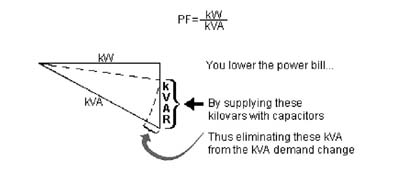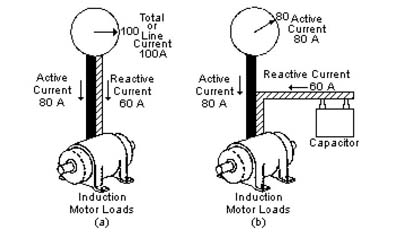# Power factor correction

As with any electrical equipment,the works of efficiency usually determine from poor to excellent.How we measure the electrical efficiency?The answer is power factor.I discussed about how to sizing capacitor for  power factor correction on my last post.

Most of electrical motor or inductive equipment powered by two types of power.First is a working power.It measure in kilo watt (kW) unit.It mean this power is actually used to operated the electrical equipment.

Second is a reactive power.It mean inductive equipment need this power to  produced the necessary flux for the operation.The measurement is the kilovar ( kVAR ) unit.

So the working power (kW) and reactive power (kVAR) combined together to make up apparent power.It measure in kilo volt ampere (KVAR) unit.That is we call power factor correction.

# kVA2 = kW2 + kVAR2## What is power factor correction?

As we known induction motors,inductive equipment, transformers and others electrical loads require magnetizing current or reactive power (kVAR) as well as actual power (kW).That why we must perform power factor correction.

To get a efficiency power usage,we need to reduce the apparent power ( kVA ) required for any given load.How to reduce the apparent power ( kVA ).

Around the world,common method for power factor correction is using the capacitor to produce reactive power ( kVAR ) and we must through or shorten the line that represents the kVAR.When capacitor supplying the right kVAR to the load, this makes the utility distribution power system more efficient, reducing cost for the utility and. The ratio of actual power ( kW ) to apparent power ( kVA ) is usually in percentage and is called power factor.eg : 80% pf = 0.80 pf

1.haitham hashim says

•lemau says

2.Sinnadurai Sripadmanaban says

Can you give a table to select size of capacitor required a)for PFC of individual motor(hp/rpm/no load current)b)for starting an induction motor of different hp/rpm/lrc

•lemau says

3.Edmund Daluddung says